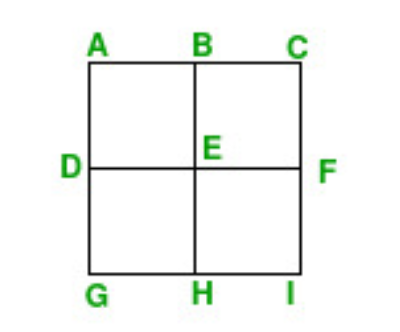Open in App
Not now

# Maximize count of unique Squares that can be formed with N arbitrary points in coordinate plane

• Last Updated : 15 Sep, 2021

Given a positive integer N, the task is to find the maximum number of unique squares that can be formed with N arbitrary points in the coordinate plane.

Note: Any two squares that are not overlapping are considered unique.

Examples:

Input: N = 9
Output: 5
Explanation:
Consider the below square consisting of N points:The squares ABEF, BCFE, DEHG, EFIH is one of the possible squares of size 1 which are non-overlapping with each other.
The square ACIG is also one of the possible squares of size 2.

Input: N = 6
Output: 2

Approach: This problem can be solved based on the following observations:

• Observe that if N is a perfect square then the maximum number of squares will be formed when sqrt(N)*sqrt(N) points form a grid of sqrt(N)*sqrt(N) and all of them are equally spaces.
• But when N is not a perfect square, then it still forms a grid but with the greatest number which is a perfect square having a value less than N.
• The remaining coordinates can be placed around the edges of the grid which will lead to maximum possible squares.

Follow the below steps to solve the problem:

1. Initialize a variable, say ans that stores the resultant count of squares formed.
2. Find the maximum possible grid size as sqrt(N) and the count of all possible squares formed up to length len to the variable ans which can be calculated by.
3. Decrement the value of N by len*len.
4. If the value of N is at least len, then all other squares can be formed by placing them in another cluster of points. Find the count of squares as calculated in Step 2 for the value of len.
5. After completing the above steps, print the value of ans as the result.

Below is the implementation of the above approach:

## C++

 // C++ program for the above approach #include using namespace std; // Function to find the maximum number// of unique squares that can be formed// from the given N pointsint maximumUniqueSquares(int N){    // Stores the resultant count of    // squares formed    int ans = 0;     // Base Case    if (N < 4) {        return 0;    }     // Subtract the maximum possible    // grid size as sqrt(N)    int len = (sqrt(double(N)));    N -= len * len;     // Find the total squares till now    // for the maximum grid    for (int i = 1; i < len; i++) {         // A i*i grid contains (i-1)*(i-1)        // + (i-2)*(i-2) + ... + 1*1 squares        ans += i * i;    }     // When N >= len then more squares    // will be counted    if (N >= len) {        N -= len;        for (int i = 1; i < len; i++) {            ans += i;        }    }     for (int i = 1; i < N; i++) {        ans += i;    }     // Return total count of squares    return ans;} // Driver Codeint main(){    int N = 9;    cout << maximumUniqueSquares(N);     return 0;}

## Java

 // Java program for the above approachimport java.io.*; class GFG { // Function to find the maximum number// of unique squares that can be formed// from the given N pointsstatic int maximumUniqueSquares(int N){    // Stores the resultant count of    // squares formed    int ans = 0;     // Base Case    if (N < 4) {        return 0;    }     // Subtract the maximum possible    // grid size as sqrt(N)    int len = (int)(Math.sqrt(N));    N -= len * len;     // Find the total squares till now    // for the maximum grid    for (int i = 1; i < len; i++) {         // A i*i grid contains (i-1)*(i-1)        // + (i-2)*(i-2) + ... + 1*1 squares        ans += i * i;    }     // When N >= len then more squares    // will be counted    if (N >= len) {        N -= len;        for (int i = 1; i < len; i++) {            ans += i;        }    }     for (int i = 1; i < N; i++) {        ans += i;    }     // Return total count of squares    return ans;} // Driver Codepublic static void main (String[] args){    int N = 9;    System.out.println( maximumUniqueSquares(N)); }} // This code is contributed by shivanisinghss2110.

## Python3

 # Python program for the above approach # for math functionimport math # Function to find the maximum number# of unique squares that can be formed# from the given N pointsdef maximumUniqueSquares(N):       # Stores the resultant count of    # squares formed    ans = 0         # Base Case    if N < 4:        return 0     # Subtract the maximum possible    # grid size as sqrt(N)    len = int(math.sqrt(N))     N -= len * len     # Find the total squares till now    # for the maximum grid    for i in range(1, len):         # A i*i grid contains (i-1)*(i-1)        # + (i-2)*(i-2) + ... + 1*1 squares        ans += i * i     # When N >= len then more squares    # will be counted    if (N >= len):        N -= len        for i in range(1, len):            ans += i     for i in range(1, N):        ans += i     # Return total count of squares    return ans # Driver Codeif __name__ == "__main__":    N = 9    print(maximumUniqueSquares(N))         # This code is contributed by rakeshsahni

## C#

 // C# program for the above approachusing System; public class GFG{     // Function to find the maximum number    // of unique squares that can be formed    // from the given N points    static int maximumUniqueSquares(int N)    {               // Stores the resultant count of        // squares formed        int ans = 0;             // Base Case        if (N < 4) {            return 0;        }             // Subtract the maximum possible        // grid size as sqrt(N)        int len = (int)(Math.Sqrt(N));        N -= len * len;             // Find the total squares till now        // for the maximum grid        for (int i = 1; i < len; i++) {                 // A i*i grid contains (i-1)*(i-1)            // + (i-2)*(i-2) + ... + 1*1 squares            ans += i * i;        }             // When N >= len then more squares        // will be counted        if (N >= len) {            N -= len;            for (int i = 1; i < len; i++) {                ans += i;            }        }             for (int i = 1; i < N; i++) {            ans += i;        }             // Return total count of squares        return ans;    }         // Driver Code    public static void Main (string[] args)    {        int N = 9;        Console.WriteLine( maximumUniqueSquares(N));         }} // This code is contributed by AnkThon

## Javascript

 

Output:

5

Time Complexity: O(sqrt(N))
Auxiliary Space: O(1)

My Personal Notes arrow_drop_up